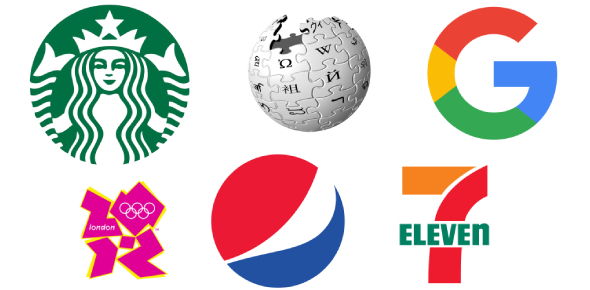# Science 8: Benchmark III-part 1 (50)

50 Questions | Total Attempts: 207SettingsCreate your own QuizReview chemical bonding, periodic table, and elements.

• 1.
Which "group" on the periodic table is the most reactive?
• A.

18

• B.

1

• C.

17

• D.

7

• 2.
What is it called when a neutral atom loses or gains an electron?
• A.

Isotope

• B.

Ion

• C.

Atom

• D.

Compound

• 3.
Which of the following is a covalent bond? Remember covalent must be a nonmetal + nonmetal or a metal + metal together, shares
• A.

KCl

• B.

N2

• C.

CO2

• D.

Ca2O

• 4.
What are two types of chemical bonding?
• A.

Polar and nonpolar

• B.

Ionic and covalent

• C.

Subscript and coefficient

• D.

Negative and positive

• 5.
Is this a balanced or unbalanced formula, Cl2 +2Na -> 2NaCl?
• A.

Maybe

• B.

Yes

• C.

No

• 6.
What is the right side of the the chemical equation called?
• A.

Reactant

• B.

Product

• C.

Coefficient

• D.

Subscript

• 7.
What is an example of a physical change?
• A.

Burning wood

• B.

Tearing paper

• C.

Tarnishing

• D.

Rusting

• 8.
What is an example of a physical change?
• A.

Ice melting

• B.

Tarnishing

• C.

Digesting food

• D.

• 9.
What is the type of nuclear reaction the releases energy or splits the nucleus?
• A.

Fusion

• B.

Fission

• C.

Energy

• D.

Breaking apart

• 10.
What is the substance called to the left of the arrow in a chemical equation?
• A.

Product

• B.

Reactant

• C.

Formula

• D.

Equation

• 11.
What does the Law of Conservation of Matter state?
• A.

Matter can be destroyed, but not reproduced.

• B.

Matter can neither be created nor destroyed in a chemical reaction

• C.

Matter can be created.

• D.

Matter can be created and destroyed in a chemical reaction.

• 12.
What does an object's in "motion" depend upon?
• A.

Velocity

• B.

Mass

• C.

Mass and velocity

• D.

Volume

• 13.
What is the pH of pure water ?
• A.

1

• B.

14

• C.

7

• D.

4

• 14.
What is an "orderly arrangement" of molecules found in a solid as a diamond or ice?
• A.

Element

• B.

Atom

• C.

Crystal

• D.

Compound

• 15.
How many of each element does rubbing alcohol, C3H7OH, contain?
• A.

C=3 H=7 O=1

• B.

C=1 H=2 O=1

• C.

C=3 H=8 O=1

• D.

C=10 H=1 O=1

• 16.
What does a spring scale measure?
• A.

Inertia

• B.

Force

• C.

Work

• D.

Motion

• 17.
What is the temperature when there is no molecular motion?
• A.

0 degrees Celsius

• B.

273 degree Kelvin

• C.

Absolute zero

• D.

32 degrees Fahrenheit

• 18.
Which changes on the moon, your weight or mass?
• A.

Mass

• B.

Weight

• C.

Weight and mass

• D.

Neither

• 19.
What would be the best unit of measurement to use when measuring a pencil in science?
• A.

Inches

• B.

Centimeter

• C.

Milligram

• D.

Kilometer

• 20.
Which of the following is a compound?
• A.

N2

• B.

Cl2

• C.

H2O

• D.

He

• 21.
What does the neutralization process make when an acid and base combines?
• A.

Acid

• B.

Base

• C.

Salt and water

• D.

Gas

• 22.
What direction does heat move?
• A.

From lower temperature to higher temperature

• B.

From higher temperature to lower temperature

• C.

Slowly absorbs

• D.

Slowly decreases from lower to higher

• 23.
How does salt melt ice on a sidewalk?
• A.

Salt makes the water evaporate

• B.

Salt heats up when the sun shines on it

• C.

Salt raises temperature above 0 degrees Celsius

• D.

Salt lowers the freezing point of water below 0 degrees Celsius

• 24.
What is it called when liquid changes to a solid?
• A.

Condensation

• B.

Evaporation

• C.

Boiling

• D.

Freezing

• 25.
What is the amount of work done in a period amount of time?
• A.

Power

• B.

Force

• C.

Motion

• D.

Velocity

Related TopicsBack to top
×

Wait!
Here's an interesting quiz for you.